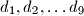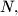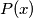### IMO Shortlist 2011 problem N2

Kvaliteta:
Avg: 0,0
Težina:
Avg: 6,0
Consider a polynomial$P(x) = \prod^9_{j=1}(x+d_j),$ where$d_1, d_2, \ldots d_9$ are nine distinct integers. Prove that there exists an integer$N,$ such that for all integers$x \geq N$ the number$P(x)$ is divisible by a prime number greater than 20.

Proposed by Luxembourg
Izvor: Međunarodna matematička olimpijada, shortlist 2011